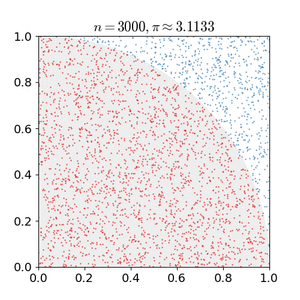# Monte Carlo algorithm facts for kids

Kids Encyclopedia Facts
(Redirected from Monte-Carlo algorithm)Monte Carlo method applied to approximating the value of π. After placing 30000 random points, what is the probability that the estimate for π is within 0.07% of the actual value?

A Monte Carlo algorithm is an algorithm for computers. It is used to simulate the behaviour of other systems. It is not an exact method, but a heuristical one. Usually it uses randomness and statistics to get a result.

It is a computation process that uses random numbers to produce an outcome(s). Instead of having fixed inputs, probability distributions are assigned to some or all of the inputs. This will generate a probability distribution for the output after the simulation is run.

For example, a Monte Carlo algorithm can be used to estimate the value of π. The amount of area within a quarter-circle of radius 1 depends on the value of π. The probability that a randomly-chosen point will lie in that quarter-circle depends on the area of the circle. If points are placed randomly in a square with sides of length 1, the percentage of points that fall within a quarter-circle of radius 1 will depend on the value of π. A Monte Carlo algorithm would randomly place points in the square and use the percentage of points falling inside of the circle to estimate the value of π.This is an effective way for making approximations.

In modern communications systems, the quality of information exchange is determined by the presence of noise in the channel. The major source of noise - Additive White Gaussian Noise (AWGN) being random in nature can be characterized using the Monte Carlo algorithm in simulating a Communications SystemMonte Carlo algorithm Facts for Kids. Kiddle Encyclopedia.